## Monday, 2 April 2012

### Basics of integration. Part II. Anti-derivatives

Recalling the result that we get in last section: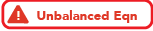That enables us to find some integrands directly instead of using some special techniques. Further more, given that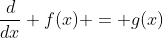, we have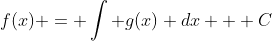. Through this section we will go through some basic properties of integration as well.

Constant rule
Example. 1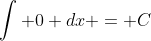, not necessarily zero. It's because y = c is the solution of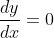. This simple example illustrates that how integrands can be solved by considering their derivatives.

Theorem. 1 (Constant rule).
Proof: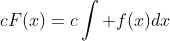and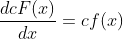, the second equation gives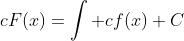, since the constant has no effect (this is an indefinite integral, constant can be grouped into the integral sign). Combining both equations give the desired result.

Polynomial
Theorem. 2 (Addition of integrands)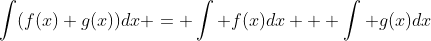Proof: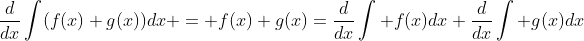which is equal to.
Integrate both sides once gives the desired result.

Theorem. 3 (Power rule)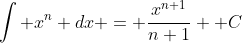for all real n except n = -1.
Proof: By power rule in the differentiation version:, integrate both sides gives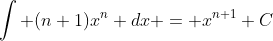which claims the desired result by constant rule.

Why does it fail at n = -1? Power rule in differentation failed in n = 0 because "this is not a polynomial, instead this is a constant so it does not work". Now for n = -1, the denominator becomes zero, making the integration result failed. However, it is clear that 1/x has an anti-derivative since it is almost everywhere continuous...

Theorem. 4 (Exception of power rule), note that there's a absolute sign here.
Proof: omitted.
Note: 1/x diverge at zero, that makes a bit a technical problem but we are not going to discuss it here. It can be solved by p.v. integration but it is far from our scope.

The trigonometrical cycle

In differentiation we know that sin x -> cos x -> - sin x  -> - cos x -> sin x is a differentiation cycle, i.e.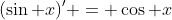,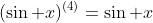(Note: such a notation with bracket is not indicating power. Instead it shows that how many times the function is to be differentiated. In this example, sin x is differentiated 4 times to get sin x again.)

Since integration is the inverse of differentiation, integration actually reverses the cycle.

Under integration, the cycle goes like sin x -> - cos x -> - sin x -> cos x -> sin x. It is exactly the reversed version of differentiation cycle of trigonometric functions. Readers should realize the fact that integration is finding the anti-derivative of a function, hence it must be the reversed cycle of the differentiation ones.

Practical example

In this section we know nothing about chain rule, substituion or other technique yet, so the main point is that you should identify and separate each term and integrate each of them by power rule or trigonometrical cycle.

Example. 2 Evaluate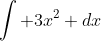.
Solution: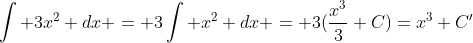. C and C' are constant so even they are added, subtracted or multiplied by a constant it doesn't matter.
Approach: you may notice that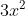is the anti-derivative of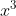, so it is expected that you can immediately get the answer.

Example. 3 Evaluate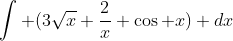.
Solution: separate each term gives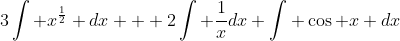Apply power rule and trigonometric cycle yields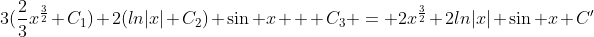Again note that in the final answer only one constant left.

Such skills are very basic and its difficulty grows like geometric series in later stage, so basic skills should be treated seriously and mass exercises are adviced.